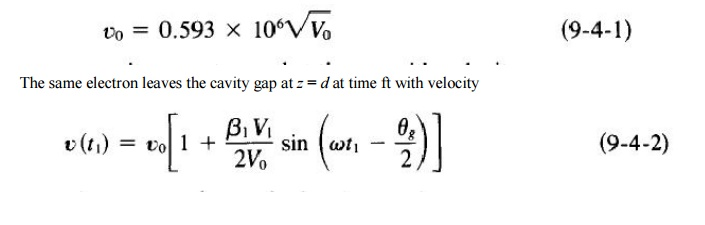Home | | RF and Microwave Engineering | Reflux Klystron

# Reflux Klystron

If a fraction of the output power is fed back to the input cavity and if the loop gain has a magnitude of unity with a phase shift of multiple 27T, the klystron will oscillate.

REFLUX KLYSTRON

If a fraction of the output power is fed back to the input cavity and if the loop gain has a magnitude of unity with a phase shift of multiple 27T, the klystron will oscillate. However, a two-cavity klystron oscillator is usually not constructed because, when the oscillation frequency is varied, the resonant frequency of each cavity and the feedback path phase shift must be readjusted for a positive feedback.

The reflex klystron is a single-cavity klystron that overcomes the disadvantages of the twocavity klystron oscillator. It is a low-power generator of 10 to 500-mW output at a frequency range of I to 25 GHz. The efficiency is about 20 to 30%. This type is widely used in the laboratory for microwave measurements and in microwave receivers as local oscillators in commercial, military, and airborne Doppler radars as well as missiles.

The theory of the two-cavity klystron can be applied to the nalysis of the reflex klystron with slight modification. A schematic diagram of the reflex klystron is shown in Fig. 9-4-1.

The electron beam injected from the cathode is first velocity-modulated by the cavity-gap voltage. Some electrons accelerated by the accelerating field enter therepeller space with greater velocity than those with unchanged velocity. Some electrons decelerated by the retarding field enter the repeller region with less velocity.

All electrons turned around by the repeller voltage then pass through the cavity gap in bunches that occur once per cycle. On their return journey the bunched electrons pass through the gap during the retarding phase of the alternating field and give up their kinetic energy to the electromagnetic energy of the field in the cavity. Oscillator output energy is then taken from the cavity. The electrons are finally collected by the walls of the cavity or other grounded metal parts of the tube. Figure 9-4-2 shows an Applegate diagram for the 1~ mode of a reflex klystron.

Velocity Modulation

The analysis of a reflex klystron is similar to that of a two-cavity klystron. For simplicity, the effect of space-charge forces on the electron motion will again be neglected. The electron entering the cavity gap from the cathode at z = 0 and time to is assumed to have uniform velocityThis expression is identical to Eq. (9-2-17), for the problems up to this point are identical to those of a two-cavity klystron amplifier. The same electron is forced back to the cavity z = d and time tz by the retarding electric field E, which is given byThis retarding field E is assumed to be constant in the z direction. The force equation for one electron in the repeller region iswhere E = - VY is used in the z direction only, Yr is the magnitude of the repeller voltage, and I Yt sin wt I ~ (Yr + Yo) is assumed. Integration of Eq. (9-4-4) twice yieldst0 time for electron entering cavity gap at z = 0

t 1 time for same electron leaving cavity gap at z = d time for same electron returned by retarding field

z = d and collected on walls of cavityStudy Material, Lecturing Notes, Assignment, Reference, Wiki description explanation, brief detail
RF and Microwave Engineering : Microwave Tubes and Measurements : Reflux Klystron |